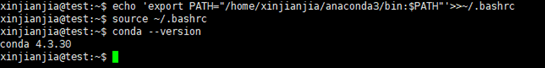# anaconda配置深度学习环境

## 第二步：安装 Anaconda

1.cd到anaconda安装包目录下，安装anaconda：

bash Anaconda3-5.0.1-Linux-x86_64.sh

2.按enter浏览完协议以后，输入yes同意协议（注意再选择安装路径的时候，按enter即可安装在默认目录下，不要再输入yes~，否则就安装在yes目录下了~ T_T）

3.运行conda指令，此时可能提示找不到conda指令，使用指令（xxx为自己的用户名）：

echo 'export PATH="/home/xxx/anaconda3/bin:\$PATH"'>>~/.bashrc
source ~/.bashrc## 第三步：创建隔离环境

conda create -n py3.7 python=3.7


## 第四步：安装 TensorFlow-GPU

conda activate py3.6

conda install tensorflow-gpu

conda install numpy, scipy, matplotlib, pandas,scikit-learn,scikit-image

## 第五步：测试一下代码

import tensorflow as tf
import numpy as np

# 使用 NumPy 生成假数据(phony data), 总共 100 个点.
x_data = np.float32(np.random.rand(2, 100)) # 随机输入
y_data = np.dot([0.100, 0.200], x_data) + 0.300

# 构造一个线性模型
#
b = tf.Variable(tf.zeros())
W = tf.Variable(tf.random_uniform([1, 2], -1.0, 1.0))
y = tf.matmul(W, x_data) + b

# 最小化方差
loss = tf.reduce_mean(tf.square(y - y_data))
train = optimizer.minimize(loss)

# 初始化变量
init = tf.initialize_all_variables()

# 启动图 (graph)
sess = tf.Session()
sess.run(init)

# 拟合平面
for step in range(0, 201):
sess.run(train)
if step % 20 == 0:
print (step, sess.run(W), sess.run(b))

# 得到最佳拟合结果 W: [[0.100  0.200]], b: [0.300]